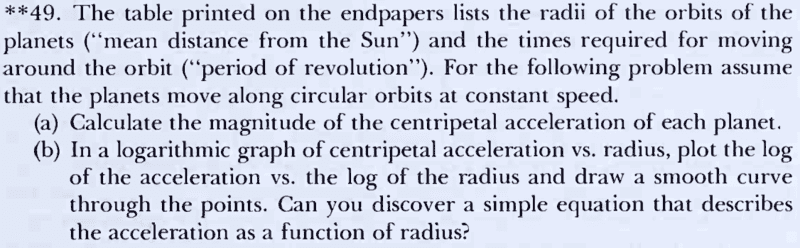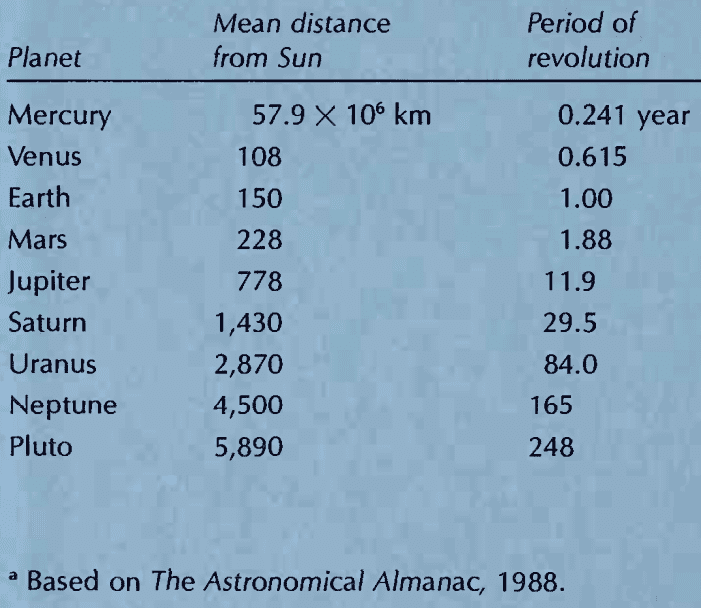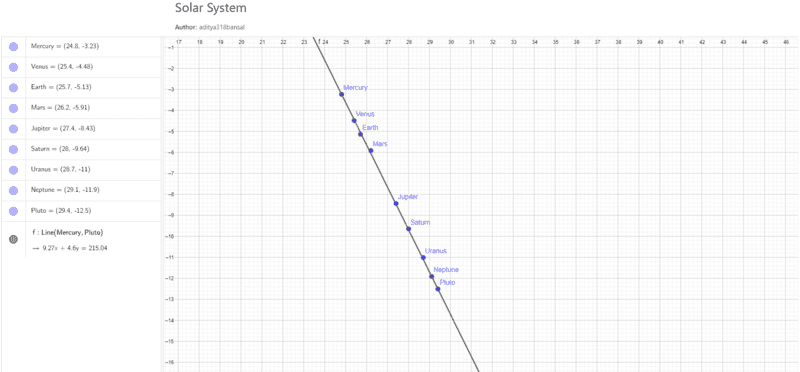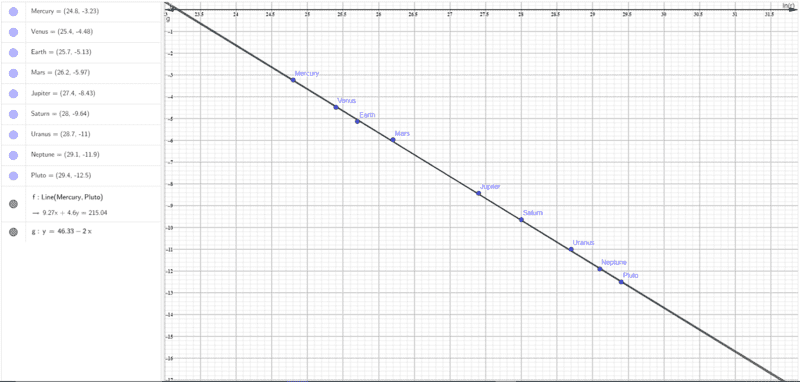# The centripetal acceleration of the planets in our solar system

• I
brochessproRelevant formulae:-
Angular velocity in uniform circular motion ##=## ##\omega## ##=## ##\frac {2\pi} t##, where ##t## is the time taken to complete one revolution.
Centripetal acceleration in uniform circular motion ##=## ##a## ##=## ##\omega^2r##, where ##r## is the radius of the circular path.

I solved both the problems. I plotted the graph in GeoGebra and obtained the following.It looks cool, even if I do say so myself.

Note that I took the natural logarithm of the obtained results, and I converted the quantities to their SI counterparts and plotted the graph.
##ln(r)## is on the X axis where ##r## is in m, and ##ln(a)## is on the Y axis where ##a## is in ms-2. A distance of 1 on the graph equals 1 unit.

My question is, why does this happen. Why do we get a straight line on joining the points? Could someone please explain how this wonderful phenomenon occurs? I could give you any other data you may require about how I made this graph. Thank you.

P.S. The book from where this problem is taken:-
Ohanian, Hans C. Physics. Vol. 1. 2nd ed., expanded. New York, NY: Norton, 1989. ISBN: 9780393957501.
http://www.amazon.com/exec/obidos/ASIN/0393957500/ref=nosim/mitopencourse-20

Latest edition:-
Ohanian, Hans C., and John T. Markert. Physics for Engineers and Scientists. Vol. 1. 3rd ed. New York, NY: Norton, 2007. ISBN: 9780393930030.
http://www.amazon.com/exec/obidos/ASIN/0393930033/ref=nosim/mitopencourse-20

Gold Member
2022 Award
Just calculate the centripetal force given that it is due to the gravitational force (for simplicity you can assume the Sun to be at rest with the planets orbiting around, because the Sun is so much heavier than all the planets in our solar system). Then you find a relation between ##r## and the acceleration.

brochesspro
Just calculate the centripetal force given that it is due to the gravitational force (for simplicity you can assume the Sun to be at rest with the planets orbiting around, because the Sun is so much heavier than all the planets in our solar system). Then you find a relation between ##r## and the acceleration.
The relation between ##r## and ##a## is already established.
Centripetal acceleration in uniform circular motion = a = ω2r, where r is the radius of the circular path.

My question is, what is the logic behind the formation of the line. Or maybe, this is the way I should put it, how do we analytically(not graphically) figure out the relation between the ##ln(r)## and the ##ln(a)## if we do not know that they will form a straight line, or, if need be, maybe we got a straight line after plotting the graph, and have to figure out the relation between the quantities, given that you already know that they form a straight line from the graph that you plotted.

Gold Member
2022 Award
Just calculate ##\ln[a(r)/a_0]##, where ##a_0## is an arbitrary constant of the dimension of an acceleration (there can never be a dimensionful argument in an ln!). How looks the graph when drawing this as a function of ##\ln(r/r_0)##, where ##r_0## is an arbitrary constant of the dimension of a length.

brochesspro
But then, there are too many variables. The equation I got is:- $$ln\left( \frac a {a_0} \right) = m ln\left( \frac r {r_0} \right) + c$$.
The variables I get are ##a_0##, ##m##, ##r_0##, and ##c##. How do I solve for these variables, assuming I input one of the values for ##a## and ##r## I already have.

brochesspro
But then, there are too many variables. The equation I got is:- $$ln\left( \frac a {a_0} \right) = m ln\left( \frac r {r_0} \right) + c$$.
The variables I get are ##a_0##, ##m##, ##r_0##, and ##c##. How do I solve for these variables, assuming I input one of the values for ##a## and ##r## I already have.
Also, ##m## is the slope and ##c## is the Y intercept.

Homework Helper
Also, ##m## is the slope and ##c## is the Y intercept.
Looking at the graph, can you read off m?

Now, suppose that acceleration is inversely proportional to the square of radius. That is, ##a = \frac{k}{r^2}## for some constant k.

Take the log of both sides. What do you get?

brochesspro
Looking at the graph, can you read off m?
I did not understand this part.
Now, suppose that acceleration is inversely proportional to the square of radius. That is, a=kr2 for some constant k.

Take the log of both sides. What do you get?
It is directly proportional to the radius but OK. I get $$ln(a) = ln(k) - 2 ln(r)$$.
Am I right? See you in 9 hours.

Last edited:
brochesspro
Looking at the graph, can you read off m?
If you mean to determine the slope, then it is $$\frac {-9.27} {4.6} \approx -2.02$$.
Am I right?

•vanhees71
Homework Helper
It is directly proportional to the radius but OK. I get $$ln(a) = ln(k) - 2 ln(r)$$.
Which means that the slope of ##\ln(a)## when graphed against ##\ln(r)## would be what?

•vanhees71
brochesspro
Which means that the slope of ##\ln(a)## when graphed against ##\ln(r)## would be what?
It would be ##-2##. Am I right?

Last edited:
•jbriggs444 and vanhees71
brochesspro
It would be ##-2##. Am I right?
So, how do I proceed from here? Going by your equation I get $$ln(a) = ln(\omega^2r) = 2 ln(\omega) + ln(r)$$.
This means that the slope of the graph obtained is 1(it is not) and the Y-intercept is a function of ##\omega## which is variable. Kindly explain, @jbriggs444.

Homework Helper
So, how do I proceed from here? Going by your equation I get $$ln(a) = ln(\omega^2r) = 2 ln(\omega) + ln(r)$$.
I do not know whose equation you are talking about. You only quoted yourself, not anyone else. But let us see if we can find it or figure it out.

Ahhh, I see it.

Someone apparently started with an assertion that ##a=\omega^2r##. This is true enough. If one held ##\omega## fixed and varied ##r## alone then acceleration would be directly proportional to ##r##.

But that is not the situation we are faced with. We have a collection of planets in circular orbits about a common primary (the Sun). For such planets, there is a relationship between ##\omega## and ##r##. You cannot vary the one independently of the other.

Consider that ##a=\frac{v^2}{r}##. Would you claim that the slope of the ##\ln(a)## versus ##\ln(r)## graph is both 1 and -1?

Putting it another way, you have looked at a formula: ##2 \ln(\omega) + \ln(r)## and treated the first term as a constant independent of r. But it is not a constant independent of r. Since ##\omega## is a function of ##r##, the term is a function of ##r## and has a non-zero derivative with respect to ##r##.

brochesspro
I do not know whose equation you are talking about. You only quoted yourself, not anyone else. But let us see if we can find it or figure it out.

Ahhh, I see it.

Someone apparently started with an assertion that ##a=\omega^2r##. This is true enough. If one held ##\omega## fixed and varied ##r## alone then acceleration would be directly proportional to ##r##.

But that is not the situation we are faced with. We have a collection of planets in circular orbits about a common primary (the Sun). For such planets, there is a relationship between ##\omega## and ##r##. You cannot vary the one independently of the other.

Consider that ##a=\frac{v^2}{r}##. Would you claim that the slope of the ##\ln(a)## versus ##\ln(r)## graph is both 1 and -1?

Putting it another way, you have looked at a formula: ##2 \ln(\omega) + \ln(r)## and treated the first term as a constant independent of r. But it is not a constant independent of r. Since ##\omega## is a function of ##r##, the term is a function of ##r## and has a non-zero derivative with respect to ##r##.
Sorry, I meant to tag this message, but never mind.
Looking at the graph, can you read off m?

Now, suppose that acceleration is inversely proportional to the square of radius. That is, ##a = \frac{k}{r^2}## for some constant k.

Take the log of both sides. What do you get?
But I do not think we can take the formula of ##a = \frac {v^2} r ## as ##v## is dependent on ##r##. Even if we do take log on both sides, after simplifying(putting ##v = \omega r##) we still get this.
So, how do I proceed from here? Going by your equation I get $$ln(a) = ln(\omega^2r) = 2 ln(\omega) + ln(r)$$.
This means that the slope of the graph obtained is 1(it is not) and the Y-intercept is a function of ##\omega## which is variable. Kindly explain, @jbriggs444.
How do I proceed? I guess I should consider acting upon this message.
Just calculate ##\ln[a(r)/a_0]##, where ##a_0## is an arbitrary constant of the dimension of an acceleration (there can never be a dimensionful argument in an ln!). How looks the graph when drawing this as a function of ##\ln(r/r_0)##, where ##r_0## is an arbitrary constant of the dimension of a length.
But the problem is, how do I get the four variables in the equation I got.
But then, there are too many variables. The equation I got is:- $$ln\left( \frac a {a_0} \right) = m ln\left( \frac r {r_0} \right) + c$$.
The variables I get are ##a_0##, ##m##, ##r_0##, and ##c##. How do I solve for these variables, assuming I input one of the values for ##a## and ##r## I already have.
Again, what do I do? It is alright if you are not able to help as I am only asking this to satiate my curiosity and this is not a part of the problem. Thank you. See you in about 11 hours.

Gold Member
2022 Award
I think it's time to answer the question now, because I've the impression you get more and more confused by trying to get it out yourself.

All you need to answer your question, is Newton's 2nd Law and the formula for the gravitational force between Sun and the planets. We can consider the Sun as fixed in the origin of our reference frame, because it is much heavier than all the planets. Then the equation of motion reads
$$m \vec{a}=- \frac{G m M}{r^2} \frac{\vec{r}}{r}.$$
Now you can also consider the planets running on circles (which is not too bad an approximation for the planets in our solar system. With the given distances of the planets from the Sun and their periods of evolution you can calculate the acceleration
$$a=\omega^2 r=(2 \pi/T)^2 r.$$
Now you plot ##a## against ##r## in a double-logarithmic plot. From the equation of motion you get
$$a=G m M/r^2.$$
devide this by an arbitrary scale factor ##a_0## and take the logarithm. We forget for a moment the issue with the dimensionful quantities. You can just think of using standard SI units on both sides and only put the numbers of the dimensionful quantities under the logarithm. Then you get
$$\ln a =\ln(G m M/r^2)=\ln(G m M)-2 \ln r,$$
and you see that indeed in the double-logarithmic plot you expect to get a straight line with slope ##-2##, and that's indeed what you approximately get.

Another important thing you can immediately learn from this ideas is Kepler's 3rd Law for the special case of circular orbits:
$$a=\omega^2 r =\frac{G M}{r^2} \; \Rightarrow \; \omega^2 r^3 =G M=\text{const}$$
for all planets, which implies that
$$\frac{r^3}{T^2} = \frac{G M}{4 \pi^2}=\text{const},$$
and that is Kepler's 3rd Law. The cubes of the distances of the planets from the Sun go like the squares of their periods of evolution for all planets.

With a bit more math you can derive all 3 of Kepler's Laws for all planetary orbits:

(1) The orbit is an ellipse with the Sun in one of its foci.
(2) The line Sun-Planet sweeps out a constant area per unit of time.
(3) ##a^3/T^2=\text{const}## for all planets, where ##a## is the major semiaxis of the elliptical orbit.

brochesspro
Now you plot a against r in a double-logarithmic plot. From the equation of motion you get
a=GmM/r2.
Is that not the formula for the force? Could you perhaps have meant the following, $$a = \frac {GM} {r^2}$$? I see you have used the formula which I quoted while taking the logarithm as well. If you really meant that, then it does not solve the problem as there is the mass of the planets to be taken into account which is not constant.
devide this by an arbitrary scale factor a0 and take the logarithm. We forget for a moment the issue with the dimensionful quantities. You can just think of using standard SI units on both sides and only put the numbers of the dimensionful quantities under the logarithm. Then you get
ln⁡a=ln⁡(GmM/r2)=ln⁡(GmM)−2ln⁡r,
and you see that indeed in the double-logarithmic plot you expect to get a straight line with slope −2, and that's indeed what you approximately get.
But, you used the formula I wrote when you derived Kepler's third law of planetary orbits for the special case of circular objects.
Another important thing you can immediately learn from this ideas is Kepler's 3rd Law for the special case of circular orbits:
a=ω2r=GMr2⇒ω2r3=GM=const
for all planets, which implies that
r3T2=GM4π2=const,
and that is Kepler's 3rd Law. The cubes of the distances of the planets from the Sun go like the squares of their periods of evolution for all planets.
OK, now, other than the possible mistake which you made, my question is answered. I get the equation as, $$ln(a) = ln(GM) - 2 ln(r)$$. Am I right?

Thank you for the hard work you put into answering my question. It means a lot.

Last edited:
Gold Member
2022 Award
The mass of the planets drop out at this level of approximation (i.e., neglecting the motion of the Sun around the center of mass, which is justified since ##m \ll M## for all planets in our solar system. Of course, in this approximation
$$a=F/m=\frac{G M}{r^2}.$$
Sorry for the typo.

Staff Emeritus
Homework Helper
Gold Member
The relation between r and a is already established.
It is not since ##\omega## depends on ##r##. You need to compute it from Newton’s laws.

•jbriggs444
brochesspro
The mass of the planets drop out at this level of approximation (i.e., neglecting the motion of the Sun around the center of mass, which is justified since ##m \ll M## for all planets in our solar system. Of course, in this approximation
$$a=F/m=\frac{G M}{r^2}.$$
Sorry for the typo.
It is alright. Thank you for the correction. And by the way, this is the graph I get after putting this equation in the graph. Note that I calculated ##ln(GM)## by substituting the appropriate values to get the Y-intercept.
OK, now, other than the possible mistake which you made, my question is answered. I get the equation as, ln(a)=ln(GM)−2ln(r). Am I right?Pretty close, do you not think?
Thank you to all the people who have contributed to this thread. It means a lot to me, really.

•vanhees71